# Heat Exchangers (Part - 3) Chemical Engineering Notes | EduRev

## Chemical Engineering : Heat Exchangers (Part - 3) Chemical Engineering Notes | EduRev

The document Heat Exchangers (Part - 3) Chemical Engineering Notes | EduRev is a part of the Chemical Engineering Course Heat Transfer for Engg..
All you need of Chemical Engineering at this link: Chemical Engineering

8.2 Thermal design of heat exchangers

The mechanical design is done by the mechanical engineers on the inputs of chemical engineers and using the codes. The most widely used code in Tubular Exchanger Manufactures Associations (TEMA). This USA code along with ASME selection VIII (unfired pressure vessel) code is used together for the mechanical design of the heat exchanger. The Indian code for the heat exchanger design IS 4503.

Here we would discuss about the process design (or thermal design) leading to the sizing of the heat exchanger. Before understanding design steps, it is necessary to understand the following for the heat exchanger.

8.2.1 Overall heat transfer coefficient

As understood by the previous discussion that generally heat exchangers are tubular in nature (Note: we are not discussing about plate type heat exchangers). Thus we can easily find out the overall heat transfer coefficient based on our previous knowledge. Figure 8.8 shows a simplest form (double pipe heat exchanger) of tubular heat exchanger, where fluid A is being heated by fluid B in a co-current flow pattern. The inside and outside radii of the inner tube is represented ri and ro. The length of the exchanger for heat transfer is considered as L for section 1 to 2.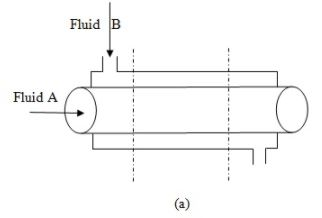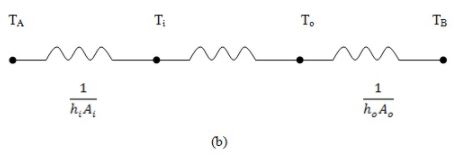Fig.8.8: (a) Schematic of a double pipe heat exchanger (b) thermal resistance network for overall heat transfer

Thus the rate of heat transfer from the hot fluid to the cold fluid will be represented by eq.8.1,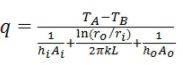(8.1)

The overall heat transfer coefficient;

Based on inside area of the inner pipe (eq.8.2)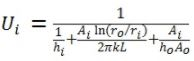(8.2)

Based on outer side area of the outer pipe (eq.8.3)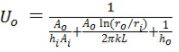(8.3)

8.2.2 Fouling factor or dirt factor

Over a time period of heat exchanger operation the surface of the heat exchanger may be coated by the various deposits present in the flow system. Moreover, the surfaces may become corroded or eroded over the time. Therefore, the thickness of the surface may get changed due to these deposits. These deposits are known as scale. These scales provide another resistance and usually decrease the performance of the heat exchangers. The overall effect is usually represented by dirt factor or fouling factor, or fouling resistance, Rf (Table 8.1) which must have included all the resistances along with the resistances due to scales for the calculation of overall heat transfer coefficient.

The fouling factor must be determined experimentally using eq. 8.4,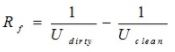(8.4)

Thus to determine the Rf, it is very important to know Uclean for the new heat exchanger. TheUclean must be kept securely to obtain the Rf, at any time of the exchanger’s life.

Table-8.1 Fouling factor of a few of the industrial fluids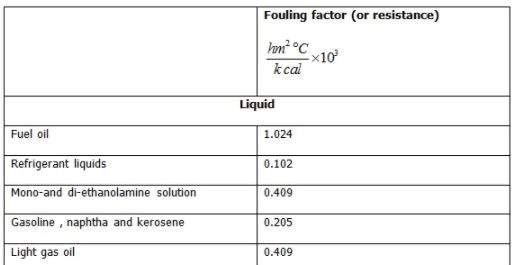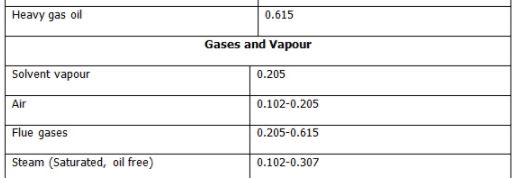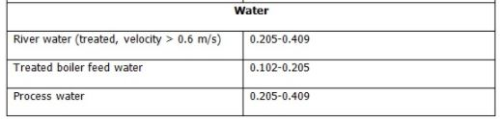8.2.3 Temperature profiles in heat exchangers

Fig. 8.9 shows the temperature profile along the length of a 1-2 exchangers and 2-4 exchangers.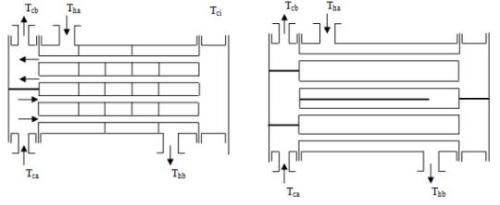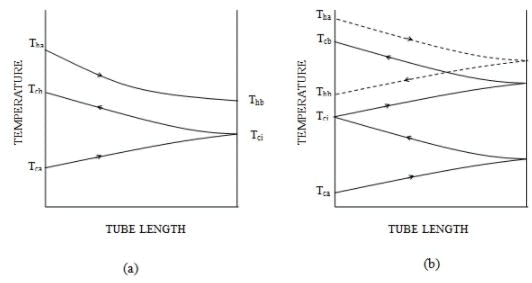Fig.8.9 Temperature-length curve corresponding to (a) 1-2 exchanger ; (b) 2-4 exchanger

The nomenclature used in the fig.8.9 is described below

Tha: Inlet temperature of hot fluid
Thb: Outlet temperature of hot fluid
Tca: Inlet temperature of cold fluid
Tcb: Outlet temperature of cold fluid
Tci: Intermediate temperature of cold fluid

In the above arrangement it is assumed that the hot fluid is flowing in the shell side and cold fluid is flowing in the tube side of the exchangers. The fig.8.9 (a) shows the 1-2 exchangers in which the hot fluid enter into the exchanger from the left side and exits from the right side. The cold fluid enters concurrently that is from the left side to the tube of the exchangers and goes up to right end of the exchangers and returns back to make two tube pass, and exits from the left end of the exchangers. The temperature profile all along the length of the exchanger is shown in the corresponding temperature length profile. Figure8.9 (b) shows the flow direction and corresponding temperature length profile for 2-4 exchangers. The shell side fluid two passes and the tube side fluid has 4-passes in the exchangers.

It can be easily understood that whenever the number of passes is more than one, the flow cannot be truly co-current or counter current. Thus it will be a mix of co-current and counter current flows in any multi pass heat exchangers.

Though the temperature profile of the hot and cold streams can be easily predictable for single pass heat exchangers but for the complex flow modes, the prediction of temperature distribution will be difficult as shown in fig.8.9. As can be seen when 1-2 exchangers was (fig.8.9 (a)) used in co-current mode, the temperature profile was given in the figure. However, if the fluid streams enter in counter current mode a temperature cross may occur sometimes. Temperature cross is described as the positive temperature difference between the cold and the hot fluid, when these fluids leave the exchangers. In that case the cold fluid will attain the maximum temperature inside the exchanger instead of at the exit (fig.8.10).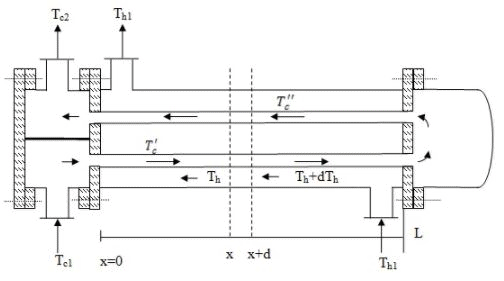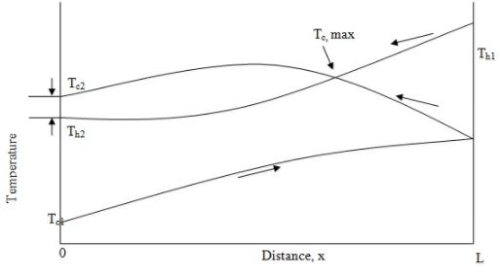Fig. 8.10: 1-2 flow pattern and temperature profile in exchanger showing cross flow

At this temperature cross, the cold fluid temperature reaches the maximum at a point inside the exchanger and not at its exits. This temperature cross point also coincides with the point of intersection of the temperature profile of the hot fluid and the co-current zone of the cold fluid. The difference (Tc2 - Th2) is called the temperature cross of the exchanger. However, if the temperature cross does not appear then the (Tc2 - Th2) is called the approach. Moreover, on careful evaluation it can be seen that for the multi shell side pass a significant length of the exchanger have cross flow pattern in the tube flow when the shell side fluid is migrating from one shell pass to another shell pass. Thus calculating heat transfer co-efficient for shell side becomes little challenging and will be explained in section 8.2.6. Although the parallel flow or counter flow arequite similar, the parallel flow and counter flow heat exchangers differ greatly in the manner in which the fluid temperatures vary as the fluid pass through. The difference can be understood in the figure 8.11.

The fig.8.11 shows an important parameter, mcp, the product of mass flow rate (m) and the specific heat, cp, of the fluids. The product mcp is called the rate of heat capacity.
The overall energy balance of the heat exchanger gives the total heat transfer between the fluids, q, expressed by eq.8.5,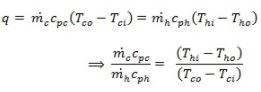(8.5)

The fig.8.11 shows the relative variation of the two fluid temperatures through the heat exchanger, which is influenced by whether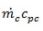is greater or less than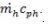.
In particular, for counter flow, examination of the sketches in fig.8.11 shows that limiting condition for maximum heat transfer is determine by whetheris greater or less than. When,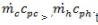the maximum possible heat transfer is determined by the fact that the hot fluid can be cooled to the temperature of the cold fluid inlet. Thus, for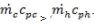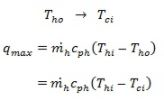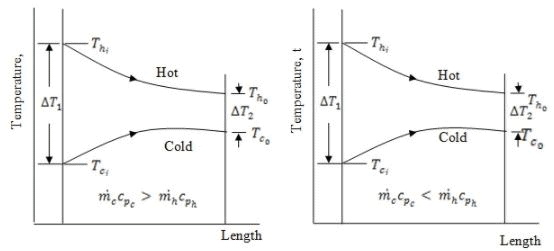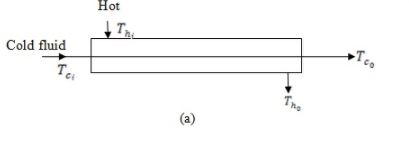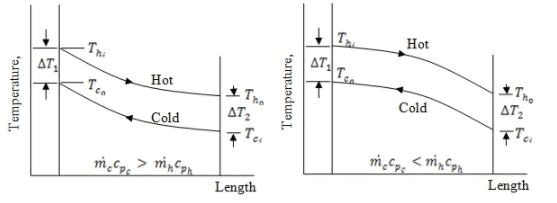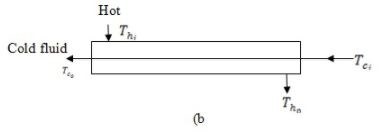Fig.8.11: Temperature profiles of (a) parallel flow, and (b) counter flow, for different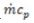inequalities

For the other case when the limit is determined as the cold fluid is heated to the inlet temperature of the hot fluid:

For,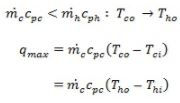Thus for the counter flow exchanger, the above two set equations show that the maximum possible heat exchanger is determined in terms of the inlet parameters. The maximum possible heat exchange may be determined (eq.8.6) by the fluid stream having low heat capacity rate;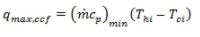(8.6)

The subscript ccf denotes counter current flow. The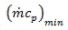is for the fluid having lower value of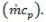In case of parallel flow, regardless of the relative sizes of the two stream the limiting heat transfer condition is determine by the fact that the two fluid streams approach the same outlet temperature. Thus,

Tho → Tco condition can be found out by the weighted average of the inlet stream.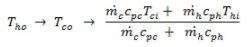Thus, the maximum possible heat transfer may be,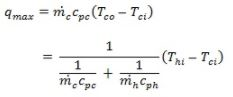or,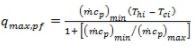(8.7)

The subscript pf represents parallel flow (co-current). From the above discussion and qmaxequations (8.6 and 8.7) it can be calculated for a given inlet conditions the counter current flow arrangement always has a better potential for heat transfer as compared to parallel flow arrangement.

Offer running on EduRev: Apply code STAYHOME200 to get INR 200 off on our premium plan EduRev Infinity!

## Heat Transfer for Engg.

46 videos|60 docs|59 tests

,

,

,

,

,

,

,

,

,

,

,

,

,

,

,

,

,

,

,

,

,

;